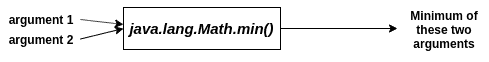# Java Math.min Function: Complete Guide

Java Math.min() method compares two numbers (can be of either int, float, double, or long type) and returns the minimum of two numbers.

## Java Math.min

Java Math.min is a built-in function that returns a minimum of two numbers. The arguments are taken in int, float, double, and long, and if both parameters passed are negative, then a number with a higher magnitude is generated as a result.

### Syntax

```public static int min(int a, int b)
public static double min(double a, double b)
public static float min(float a, float b)
public static long min(long a, long b)
```

### Parameters

Two numbers out of which the minimum is to be determined.

### Return Value

The minimum of the two arguments.

See the following figure.#### Note

1. If one argument is positive and the other is negative, then the negative argument is returned.
2. If both arguments are negative, the one with a higher magnitude is returned.
3. If one argument is positive zero, and the other is negative zero, then negative zero is returned. This is because the min() method considers negative zero strictly smaller than positive zero (unlike numerical comparison operators).
4. If either argument is NaN, then the result is NaN.

Consider the following examples.

## Comparing two int, float, double or long values, and a comparison with negative infinity.

See the following code.

```public class Example1 {
public static void main(String[] args) {
int i1 = 2;
int i2 = 3;

float f1 = 2.0f;
float f2 = 3.0f;

double d1 = 2.0;
double d2 = 3.0;

long l1 = 2000000;
long l2 = 3000000;

System.out.println(Math.min(i1, i2));
System.out.println(Math.min(f1, f2));
System.out.println(Math.min(d1, d2));
System.out.println(Math.min(l1, l2));

System.out.println(Math.min(-3.0, -3.0 / 0));
}
}
```

#### Output

```->javac Example1.java
->java Example1
2
2.0
2.0
2000000
-Infinity```

## Comparing a positive and a negative argument

```public class Example2 {
public static void main(String[] args) {
int a = 34;
int b = -45;

System.out.println(Math.min(a, b));
}
}
```

#### Output

```->javac Example2.java
->java Example2
-45
```

## Comparing two negative arguments

```public class Example3 {
public static void main(String[] args) {
int a = -34;
int b = -45;

System.out.println(Math.min(a, b));
}
}
```

#### Output

```->javac Example3.java
->java Example3
-45
```

#### Example4.java: The following example demonstrates comparing positive zero and negative zero.

```public class Example4 {
public static void main(String[] args) {
float a = -0.0f;
float b = 0.0f;

System.out.println(Math.min(a, b));
}
}
```

#### Output

```->javac Example4.java
->java Example4
-0.0
```

## Passing a NaN argument

```public class Example5 {
public static void main(String[] args) {
float a = 2f;
System.out.println(Math.min(a, 2.0 % 0));
}
}
```

#### Output

```->javac Example5.java
->java Example5
NaN
```

That’s it for this tutorial.